# Wind Support to Bird migration

Raphaël Nussbaumer1,*, Baptiste Schmid1 , Silke Bauer1 and Felix Liechti1
1 Swiss Ornithological Institute, Sempach, Switzerland
* Correspondence: raphael.nussbaumer@vogelwarte.ch
Februray 12th, 2021

## Abstract

• Bird airspeed has a significant influence on energy depletion and consequently on survival of migrant bird. In a context of climatique change, understanding how bird navigate in the air is essential (use of windspeed, altitude, spring vs autumn)
• In this study, we examine the airspeed chosen by birds using 37 weather radar in Western Europe collected over a full year.
• Althgouh large variation of groundspeed were recorded, birds flew at a relative constant airspeed between season, altitude and region.

## Introduction

How do bird compensate for wind?
• strengh and direction of wind
• prefered direction
• altitude
• time/season
• timing of deparute: are bird compensating more early in the night?
• (- specie of bird, size)

## Data

Bird density, orientation and speed for 37 weather radars in western Europe are downloaded from the ENRAM repository (ERAM, 2020) and processed as explained in Nussbaumer (2021) (https://doi.org/10.1101/2021.03.08.434434). The final dataset consists of bird density (ρ) [bird/km^3], bird flight speed following the east-west component (u) and south-north component (v) [m/s] at 15min resolutions, 200m bin altitude (0-5km) for each radar are available on zenodo (https://doi.org/10.5281/zenodo.3610184).
NNT is Normalized night time (-1: sunset to 1 sunrise):
NNT = datenum(repmat(dc(1).time',1,numel(dc))-mean(cat(3,[dc.dawn],[dc.dusk]),3)) ./ datenum([dc.dawn]-[dc.dusk])*2;
Day grouping
[G_day,day_num]=findgroups(datenum(dateshift(dc(1).time,'start','day','nearest')));
We convert the structure data to matrix to ease of use later. At the same time, we do the following (1) convert orientation and speed into vector (NS, EW componenents as complex value), (2) remove data below ground level and most importantly (3) remove all interpolated data because we are not interested in the spatio-temporal consistency of the data, but rather limiting our analysis to the most only original data.
gs=nan(numel(dc(1).time), numel(dc(1).alt), numel(dc)); dens=gs; sd_vvp=gs;
for i_d=1:numel(dc)
% data to keep
keep = true(size(dc(i_d).dens4));
keep(repmat(1:dc(i_d).scatter_lim,numel(dc(1).time),1))=false; % remvove below ground
keep(isnan(dc(i_d).dens3) | dc(i_d).dens3==0)=false; % remove when not interpolated
keep(NNT(:,i_d)<-1 & NNT(:,i_d)>1,:) = false;
% density
tmp = dc(i_d).dens4; tmp(~keep)=nan;
dens(:,:,i_d) = tmp;
% variance of speed
tmp = dc(i_d).sd_vvp; tmp(~keep)=nan;
sd_vvp(:,:,i_d) = tmp;
% groundspeed vector
keep(isnan(dc(i_d).u) | isnan(dc(i_d).v))=false; % remove when not interpolated
tmp = dc(i_d).ub + 1i*dc(i_d).vb; tmp(~keep)=nan;
gs(:,:,i_d) = tmp;
end

## Climate Reanalaysis

The U (parmID=131) and V (parmID=132) component of wind and the air temperature t (parmID=130) are downloaed from the ERA5 reanalysis (https://doi.org/10.24381/cds.bd0915c6) at pressure level (from 1000hPa to 550hPa), downloaded at the maximal resoluation (hourly and 0.25°x0.25°). All three variables are linearly interpolated (time-space 4D) at each datapoint of the weather radar data (see https://rafnuss-postdoc.github.io/BMM/2018/LiveScript/Insect_removal.html#H_38C69C30) using the barometric formula.

## Air density

Aims is to build a function converting airspeed into energy, to do so, we need the air density on the same data format than the other variable (density, speed etc...)We assume a constant specific gas constant
R_specific = 287.058; % J/(kg·K)
Pressure is taken from the altitude of each bin with the barometric formula.
dc_pressure = (1-dc(1).alt*2.25577*10^(-5)).^(5.25588)*101325/100;
Air density is thus:
airdens=nan(numel(dc(1).time), numel(dc(1).alt), numel(dc));
for i_d=1:numel(dc)
airdens(:,:,i_d) = repmat(dc_pressure,numel(dc(i_d).time),1)*100 ./ dc(i_d).wt /R_specific; % hPa * Pa/hPa / K * kg*K/J = Pa*kg/J = kg/m^3
end

## Windspeed

ws=nan(numel(dc(1).time), numel(dc(1).alt), numel(dc));
for i_d=1:numel(dc)
ws(:,:,i_d) = dc(i_d).ws; % windspeed vector
end

## Airspeed

Birds' groundspeed (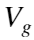) and airspeed (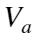) can be computed respectively with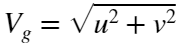and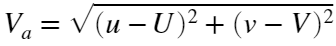.
Airspeed vector
as = gs-ws;

## Wind profit

Assuming a migration orientation of 225° (or 45°), the wind profit, quantify the wind support in the direction of migration (i.e., independant from the bird speed)
wps = cosd(45)*real(ws) + sind(45)*imag(ws);
Speed norm
v_g = abs(gs);
v_a = abs(as);
v_w = abs(ws);
Speed orientation
% angle(gs)
figure('position',[0 0 1200 300]);
subplot(1,3,1); hold on; box on;
bar(reshape(sum(sum(~isnan(dens) & dens~=0,1),2),1,[]))
bar(reshape(sum(sum(~isnan(v_g) & v_g~=0,1),2),1,[]))
subplot(1,3,2); hold on; box on;
bar(datetime(day_num,'convertfrom','datenum'), splitapply(@sum,sum(sum(~isnan(dens) & dens~=0,3),2),G_day'));
bar(datetime(day_num,'convertfrom','datenum'), splitapply(@sum,sum(sum(~isnan(v_g) & v_g~=0,3),2),G_day'));
axis tight;
xlabel('Time'); ylabel('# of Datapoint')
subplot(1,3,3); hold on; box on;
bar(dc(1).alt,sum(sum(~isnan(dens) & dens~=0,1),3))
bar(dc(1).alt,sum(sum(~isnan(v_g) & dens~=0,1),3))
xlabel('Altitude'); ylabel('# of Datapoint')
legend('Density','groundspeed')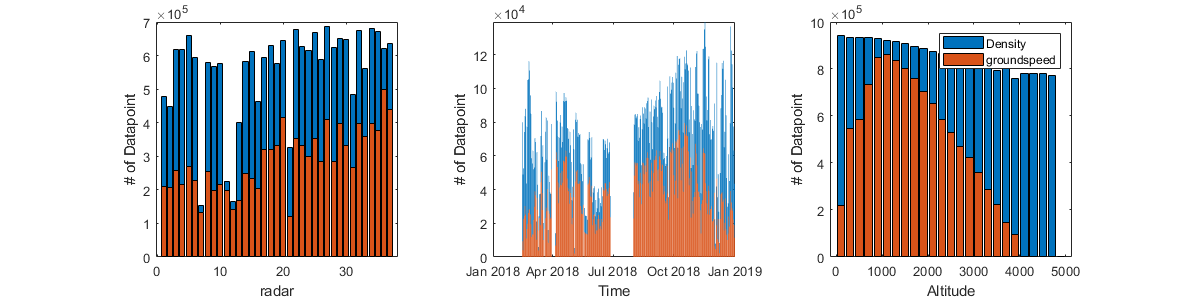Figure : Data availability measuring the total number of data point which are not NA or 0 for density and groundspeed (airspeed is same as groundspeed).
• We have much fewer datapoint of speed than density. Most of them are at high altitude >4000m, where density is weak and speed is unrealiable.
• Slightly more datapoint for German radar, but nothing important
• Annual coverage is relatively good, matching roughly the density (i.e., speed is only available when many bird are flying).

## Method

We split the dataset in over space and time.

## Split dataset : Seasonal

Over time, we define 4 periods: Early spring (March), Late spring (April), Early autumn (august-september) and late autumn (October). Note that we might want to redefine this period to match bird migration data (e.g., MTR). Here a coarse approach is to use entire month.
id_sp = dc(1).time>datetime('1-Mar-2018') & dc(1).time<datetime('1-May-2018');
id_esp = dc(1).time>datetime('1-Mar-2018') & dc(1).time<datetime('1-April-2018');
id_lsp = dc(1).time>datetime('1-April-2018') & dc(1).time<datetime('1-May-2018');
id_au = dc(1).time>datetime('1-August-2018') & dc(1).time<datetime('1-Nov-2018');
id_eau = dc(1).time>datetime('1-August-2018') & dc(1).time<datetime('1-Oct-2018');
id_lau = dc(1).time>datetime('1-Oct-2018') & dc(1).time<datetime('1-Nov-2018');
All periods combined
id_s = [id_esp; id_lsp; id_eau; id_lau];
id_s_name = {'March','April','Aug-sept.','October'};
id_s_col = [];
id_sp_col = [51 137 86]/255;
id_au_col = [229 103 7]/255;
We might also want to compare data of 2016 to see the difference.
id_2016 = dc(1).time>datetime('19-Sep-2018') & dc(1).time<datetime('9-Oct-2018');

## Split dataset: Geographic

We group radars in two groups of equal size based on their position along the general flow of migration (south-west / north east gradient with a flow orientation of 222°). The Southern group include all French radar (18) exepect frman and the northern group include all germans (16), dutch (2) and Belgium (1) radars.
id_no = [true(16,1); false(12,1); true ;false(6,1) ;true(2,1)];
id_so = ~id_no;
id_de = [false; true(15,1); false(21,1)];
id_fr = [false(16,1); true(19,1); false(2,1)];
id_sno_col = [82 88 166; 178 164 34]/255;
Illustration
w=.07; h=0.05; m =.2;
pw = @(lon) (lon-min([dc.lon]))/(max([dc.lon])-min([dc.lon]))*(1-2*m)+m;
ph = @(lat) (lat-min([dc.lat]))/(max([dc.lat])-min([dc.lat]))*(1-2*m)+m;
figure('position',[0 0 1000 1000]);
plot(coastlon,coastlat,'-k');
xlim([min([dc.lon]) max([dc.lon])])
ylim([min([dc.lat]) max([dc.lat])])
for i_d=1:numel(dc)
[esp,hsp,~,~,Mdd(i_d,1)] = histvdens(dc(i_d).dd(id_sp,:), dens(id_sp,:,i_d).*v_g(id_sp,:,i_d), 100);
[eau,hau,~,~,Mdd(i_d,2)] = histvdens(dc(i_d).dd(id_au,:), dens(id_au,:,i_d).*v_g(id_au,:,i_d), 100);
%[e2016,h2016,~,~,Mdd(i_d,3)] = histvdens(dc(i_d).dd(id_2016,:), dens(id_2016,:,i_d).*v_g(id_2016,:,i_d), 100);
subAX = axes('position',[pw(dc(i_d).lon)-w/2 ph(dc(i_d).lat)-h/2 w h]);
%tmp=round(reshape(dens(id_sp,:,i_d).*v_g(id_sp,:,i_d),1,[])); tmp(isnan(tmp)) = 0;
%polarplot(angle([Mdd(i_d,1) Mdd(i_d,1)]),[0 max(hsp)],'--','Color',id_sp_col,'LineWidth',2)
%polarplot(angle([Mdd(i_d,2) Mdd(i_d,2)]),[0 max(hau)],'--','Color',id_au_col,'LineWidth',2)
% polarplot(angle([Mdd(i_d,3) Mdd(i_d,3)]),[0 max([h2016;h2016])],'LineWidth',2)
Ax = gca; Ax.ThetaGrid = 'off'; Ax.RGrid='off'; Ax.RTickLabel = []; Ax.ThetaTickLabel = [];
title(dc(i_d).name,'Color',id_sno_col(id_no(i_d)+1,:))
end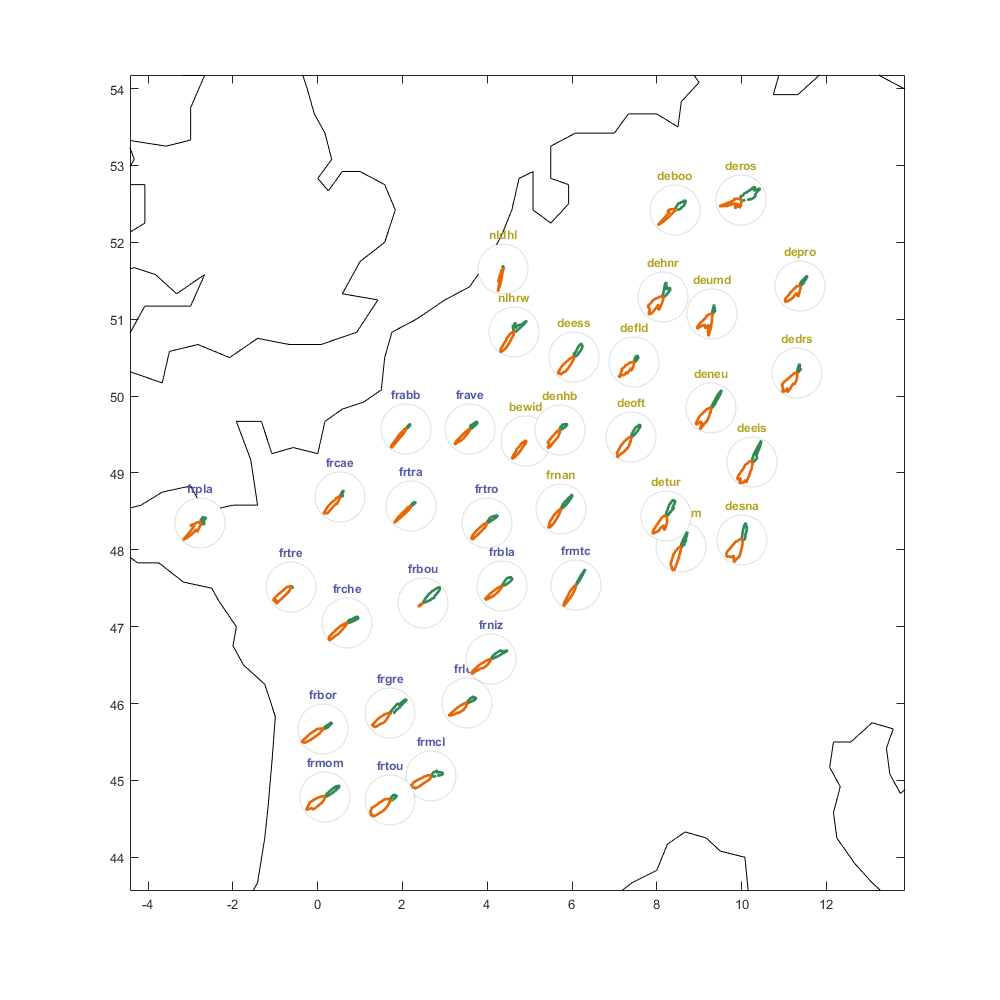Figure : Distribution of bird flight direction (groundspeed) weighted by the MTR (density x speed) for Spring (blue) and Autumn (red). Radars are grouped in the the northern (yellow) and southern (blue). Location not correct yet...

## Energy Calculation

In this section we define the energy power function, which provides the energy eng spent by a bird of mass m, wingspan B would spend flying at vt and with air density airdens. We also explore quickly the variation of optimal speed (i.e, lower energy) for different type of bird.
Parameter for energy speding
k=1.2; % [-] Induced power factor (p. 45).
% m [kg] mass of bird.
gcst = 9.81; % [ms-2] gravity constant
% Vt [m/s] true speed (bird speed-wind speed)
% B [m] Wing span.
% airdens = 1; % Air density
Sb = @(m) 0.00813*m^0.666; % [m2] body frontal area
CDb = 0.1; % [-] body drag coefficient (p. 51).
Cpro = 8.4;
Ra = 7;
Define the function of energy
f_eng = @(Vt, airdens, m, B) (2*k*(m*gcst)^2)./(Vt*pi*B.^2.*airdens) + airdens.*Vt.^3*Sb(m)*CDb/2 + Cpro/Ra*1.05*k^(3/4)*m^(3/2)*gcst^(3/2)*Sb(m)^(1/4)*CDb^(1/4)./airdens.^(1/2)/B^(3/2);
Use data from appendix of Aurbach et al., (2020) for average mass and wingspan for migrant.
Sp = table();
Sp.name = {'Willow Warbler' 'Tree Pipit' 'Common Chiffchaff' 'Spotted Flycatcher' 'Garden Warbler' 'Common Whitethroat' 'European Pied Flycatcher' 'Common Redstart' 'Wood Warbler' 'Eurasian Blackcap'}';
Sp.massmin = [8 20 6 13 16 12 9 12 7 14]';
Sp.massmax = [10 25 9 19 23 18 15 20 12 20]';
Sp.wingmin = [17 25 15 23 20 19 22 21 20 22]';
Sp.wingmax = [22 27 21 25 24 23 24 24 24 24]';
Sp.abondance = [27.5 12.7 11.3 8.7 7.3 7.1 6.8 6.6 6.1 5.9]';
Compute average mass and wingspan
Sp.massmean = (Sp.massmin+Sp.massmax)/2/1000; % [m]
Sp.wingmean = (Sp.wingmin+Sp.wingmax)/2/100; % [kg]
Sort the table by mass
Sp=sortrows(Sp,'massmean')
Sp = 10×8 table
namemassminmassmaxwingminwingmaxabondancemassmeanwingmean
1'Common Chiffchaff'69152111.30000.00750.1800
2'Willow Warbler'810172227.50000.00900.1950
3'Wood Warbler'71220246.10000.00950.2200
4'European Pied Flycatcher'91522246.80000.01200.2300
5'Common Whitethroat'121819237.10000.01500.2100
6'Spotted Flycatcher'131923258.70000.01600.2400
7'Common Redstart'122021246.60000.01600.2250
8'Eurasian Blackcap'142022245.90000.01700.2300
9'Garden Warbler'162320247.30000.01950.2200
10'Tree Pipit'2025252712.70000.02250.2600
Compute the weighted average (accounting for abundance of each species
B_avg = sum(Sp.wingmean.*Sp.abondance/100);
m_avg = sum(Sp.massmean.*Sp.abondance/100);
Energy as a function of species mass and wingspan
vt=1:0.01:20;
p=nan(size(Sp,2),1);
figure('position',[0 0 800 400]); hold on; box on;
for i=1:size(Sp,2)
Pvt = f_eng(vt, 1, Sp.massmean(i), Sp.wingmean(i));
[~, tmp] = min(Pvt);
p(i)=plot(vt, Pvt,'LineWidth',2,'displayname',[Sp.name{i} ' : ' num2str(vt(tmp)) ,' m/s' ]);
scatter(vt(tmp),min(Pvt),'or','filled')
end
legend(p); xlabel('Bird airspeed [m/s]'); ylabel('Mechanical power of the Bird [Watt=J/s]');
title('Energy curve assuming air dens=1')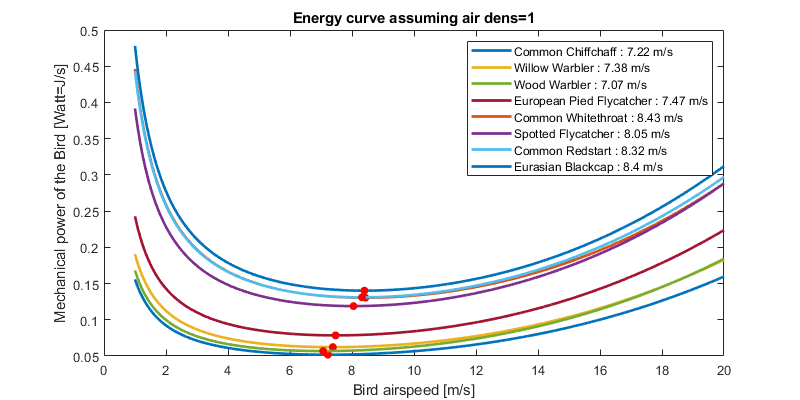The variation of optimal speed does not seem huge with these bird (7.2 - 8.4 m/s). But, we are missing some bigger bird (e.g., thrush).
Energy as a function of air density
figure('position',[0 0 600 200]); histogram(airdens); xlabel('Air density'); ylabel('PDF of dataset')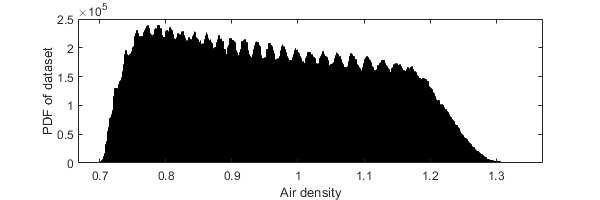Air density presents in the database.
figure('position',[0 0 800 400]); hold on; box on;
[~, tmp] = min(Pvt);
p(i)=plot(vt, Pvt,'LineWidth',2,'displayname',['air dens=' num2str(ad(i)) ' : ' num2str(vt(tmp)) 'm/s']);
scatter(vt(tmp),min(Pvt),'or','filled')
end
legend(p); xlabel('Bird airspeed [m/s]'); ylabel('Mechanical power of the Bird [Watt=J/s]');
title(['Energy curve assuming average size bird (m=' num2str(m_avg) 'kg, B=' num2str(B_avg) 'm)'])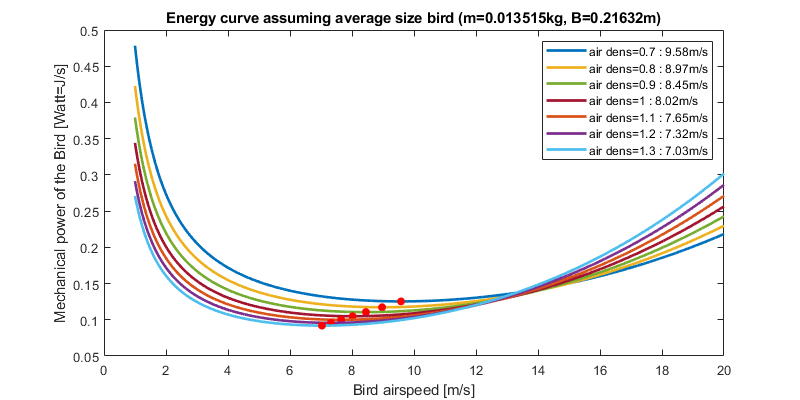Note that air density plays also a role in the optimale speed of bird, whithin the variation of the airdensity observed (computed from ERA dataset).
[V,A] = meshgrid(1:20,0.9:0.02:1.2);
E = f_eng(V, A, m_avg,B_avg);
lim = 50;
tmp = [v_a(dens>lim) airdens(dens>lim) dens(dens>lim)];
[~,idx] = sort(tmp(:,3)); % sort just the first column
tmp = tmp(idx,:);
figure('position',[0 0 1200 500]);
subplot(1,2,1)
scatter(tmp(:,1),tmp(:,2),[],tmp(:,3),'filled')
xlabel('airspeed [m/s]'); ylabel('air density [-]');
c=colorbar; c.Label.String='Bird density [bird/km^2]';
xlim([0 20]); ylim([0.9 1.25]);
subplot(1,2,2);
imagesc(V(1,:),A(:,1),E);
ax = gca; ax.YDir = 'normal';
xlim([0 20]); ylim([0.9 1.25]);
xlabel('airspeed [m/s]'); ylabel('air density [-]');
c=colorbar; c.Label.String='Mechanical power of the Bird [Watt=J/s]';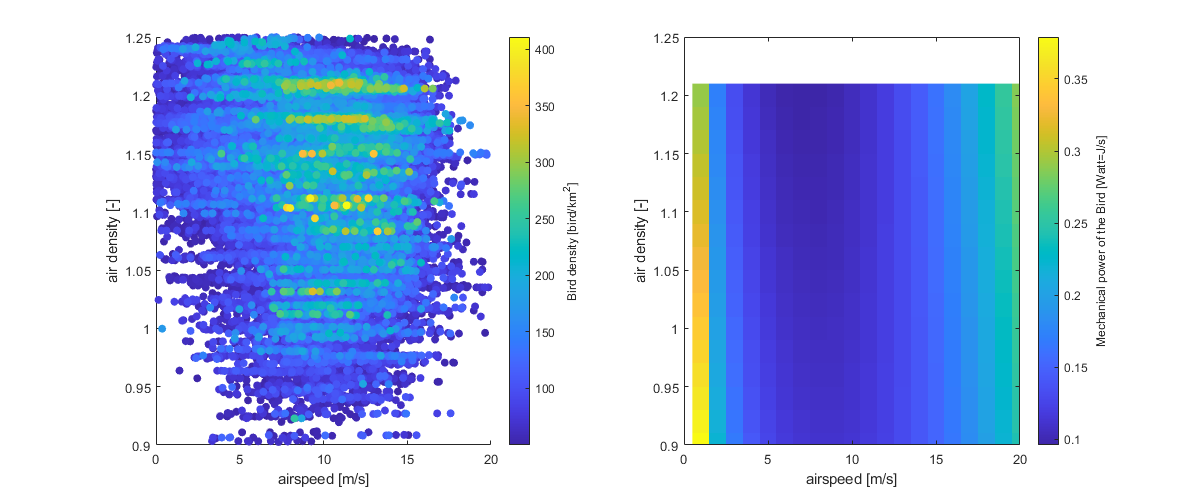In this figure, I compare the airspeed/airdensity usage of bird with the optimal according to mechanical theory. Left subplot shows the actually usage (warmer color=more bird), and right panel shows the optimal for an average sized bird (colder cooler=more optimal).
• Air density does not playa big role
• Bird should really not fly lower than 3m/s, yet we do observed some airspeed very low.
• Generally, highest density are present with airspeed slightly higher than theory (9-12m/s vs 7-10 m/s).
Compute the energy for the entire dataset assuming an averaged size bird.
eng = f_eng(v_a, airdens, m_avg, B_avg);

## Reshape data as table

Reshape into table to reduce space and help in the analysis
% [tmp_time, tmp_alt, tmp_radar] = meshgrid(dc(1).time,dc(1).alt,1:37);
% T

## Air vs Ground Overall

First, I'm looking at confirming basic trend which are already known to check that all the methodolgy and dataset are correct.
figure('position',[0 0 1000 300]);
subplot(1,2,1); hold on;
[b,h,Mg,Sg] = histvdens(v_g, dens);
bar(b,h,'FaceAlpha',0.6);
[b,h,Ma,Sa] = histvdens(v_a, dens);
bar(b,h,'FaceAlpha',0.6);
%plot(0:30,normpdf(0:30,Mg,Sg)./max(normpdf(0:30,Mg,Sg))*max(tmp3),'-r');
%plot(0:30,normpdf(0:30,M,Sa)./max(normpdf(0:30,M,Sa))*max(tmp3),'-r');
legend(['Groundspeed. M=' num2str(Mg,3) ' ; S=' num2str(Sg,3)], ['Airspeed. M=' num2str(Ma,3) ' ; S=' num2str(Sa,3)])
xlabel('Bird speed [m/s]'); box on
ylabel('Sum of bird density [bird/km^3]'); xlim([0 30]);
subplot(1,2,2); hold on;
[~, id] = sort(dens(:)); id2 = id(dens(id)>5); % only keep density >5bird/km^2 for computing reason
scatter(v_a(id2),v_g(id2),[],dens(id2),'filled'); axis tight equal;
plot([0 40],[0 40],'k','LineWidth',2); box on
xlabel('airspeed [m/s]'); ylabel('groundspeed [m/s]');
c=colorbar; c.Label.String='Bird density [bird/km^2]';